## Round Robin Scheduling-

In Round Robin Scheduling,

• CPU is assigned to the process on the basis of FCFS for a fixed amount of time.
• This fixed amount of time is called as time quantum or time slice.
• After the time quantum expires, the running process is preempted and sent to the ready queue.
• Then, the processor is assigned to the next arrived process.
• It is always preemptive in nature.

 Round Robin Scheduling is FCFS Scheduling with preemptive mode.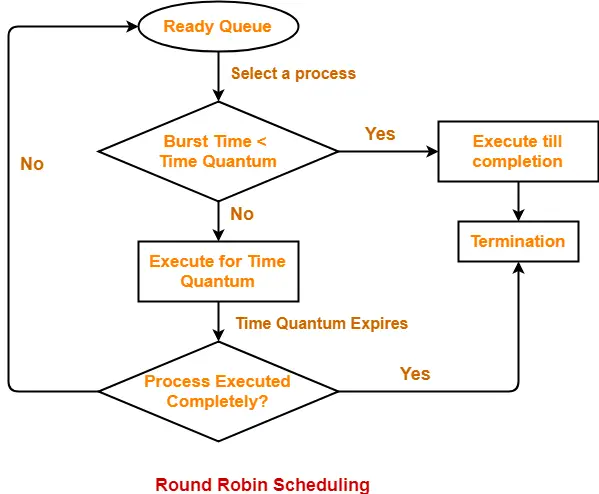• It gives the best performance in terms of average response time.
• It is best suited for time sharing system, client server architecture and interactive system.

• It leads to starvation for processes with larger burst time as they have to repeat the cycle many times.
• Its performance heavily depends on time quantum.
• Priorities can not be set for the processes.

## Important Notes-

### Note-01:

With decreasing value of time quantum,

• Number of context switch increases
• Response time decreases
• Chances of starvation decreases

Thus, smaller value of time quantum is better in terms of response time.

### Note-02:

With increasing value of time quantum,

• Number of context switch decreases
• Response time increases
• Chances of starvation increases

Thus, higher value of time quantum is better in terms of number of context switch.

### Note-03:

• With increasing value of time quantum, Round Robin Scheduling tends to become FCFS Scheduling.
• When time quantum tends to infinity, Round Robin Scheduling becomes FCFS Scheduling.

### Note-04:

• The performance of Round Robin scheduling heavily depends on the value of time quantum.
• The value of time quantum should be such that it is neither too big nor too small.

## Problem-01:

Consider the set of 5 processes whose arrival time and burst time are given below-

 Process Id Arrival time Burst time P1 0 5 P2 1 3 P3 2 1 P4 3 2 P5 4 3

If the CPU scheduling policy is Round Robin with time quantum = 2 unit, calculate the average waiting time and average turn around time.

## Solution-

### Gantt Chart-

P5, P1, P2, P5, P4, P1, P3, P2, P1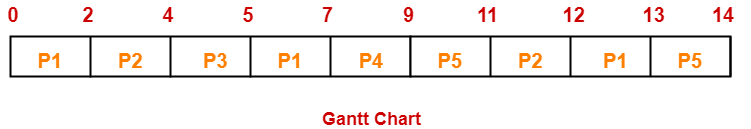Now, we know-

• Turn Around time = Exit time – Arrival time
• Waiting time = Turn Around time – Burst time

Also read- Various Times of Process

 Process Id Exit time Turn Around time Waiting time P1 13 13 – 0 = 13 13 – 5 = 8 P2 12 12 – 1 = 11 11 – 3 = 8 P3 5 5 – 2 = 3 3 – 1 = 2 P4 9 9 – 3 = 6 6 – 2 = 4 P5 14 14 – 4 = 10 10 – 3 = 7

Now,

• Average Turn Around time = (13 + 11 + 3 + 6 + 10) / 5 = 43 / 5 = 8.6 unit
• Average waiting time = (8 + 8 + 2 + 4 + 7) / 5 = 29 / 5 = 5.8 unit

## Problem-02:

Consider the set of 6 processes whose arrival time and burst time are given below-

 Process Id Arrival time Burst time P1 0 4 P2 1 5 P3 2 2 P4 3 1 P5 4 6 P6 6 3

If the CPU scheduling policy is Round Robin with time quantum = 2, calculate the average waiting time and average turn around time.

## Solution-

### Gantt chart-

P5, P6, P2, P5, P6, P2, P5, P4, P1, P3, P2, P1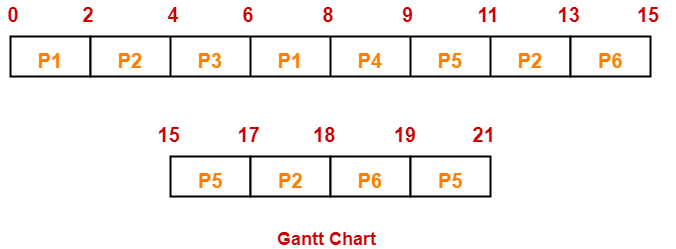Now, we know-

• Turn Around time = Exit time – Arrival time
• Waiting time = Turn Around time – Burst time

 Process Id Exit time Turn Around time Waiting time P1 8 8 – 0 = 8 8 – 4 = 4 P2 18 18 – 1 = 17 17 – 5 = 12 P3 6 6 – 2 = 4 4 – 2 = 2 P4 9 9 – 3 = 6 6 – 1 = 5 P5 21 21 – 4 = 17 17 – 6 = 11 P6 19 19 – 6 = 13 13 – 3 = 10

Now,

• Average Turn Around time = (8 + 17 + 4 + 6 + 17 + 13) / 6 = 65 / 6 = 10.84 unit
• Average waiting time = (4 + 12 + 2 + 5 + 11 + 10) / 6 = 44 / 6 = 7.33 unit

## Problem-03:

Consider the set of 6 processes whose arrival time and burst time are given below-

 Process Id Arrival time Burst time P1 5 5 P2 4 6 P3 3 7 P4 1 9 P5 2 2 P6 6 3

If the CPU scheduling policy is Round Robin with time quantum = 3, calculate the average waiting time and average turn around time.

## Solution-

### Gantt chart-

P3, P1, P4, P2, P3, P6, P1, P4, P2, P3, P5, P4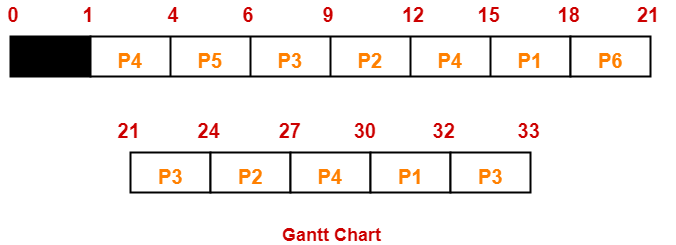Now, we know-

• Turn Around time = Exit time – Arrival time
• Waiting time = Turn Around time – Burst time

 Process Id Exit time Turn Around time Waiting time P1 32 32 – 5 = 27 27 – 5 = 22 P2 27 27 – 4 = 23 23 – 6 = 17 P3 33 33 – 3 = 30 30 – 7 = 23 P4 30 30 – 1 = 29 29 – 9 = 20 P5 6 6 – 2 = 4 4 – 2 = 2 P6 21 21 – 6 = 15 15 – 3 = 12

Now,

• Average Turn Around time = (27 + 23 + 30 + 29 + 4 + 15) / 6 = 128 / 6 = 21.33 unit
• Average waiting time = (22 + 17 + 23 + 20 + 2 + 12) / 6 = 96 / 6 = 16 unit

## Problem-04:

Four jobs to be executed on a single processor system arrive at time 0 in the order A, B, C, D. Their burst CPU time requirements are 4, 1, 8, 1 time units respectively. The completion time of A under round robin scheduling with time slice of one time unit is-

1. 10
2. 4
3. 8
4. 9

## Solution-

 Process Id Arrival time Burst time A 0 4 B 0 1 C 0 8 D 0 1

### Gantt chart-

C, A, C, A, C, A, D, C, B, A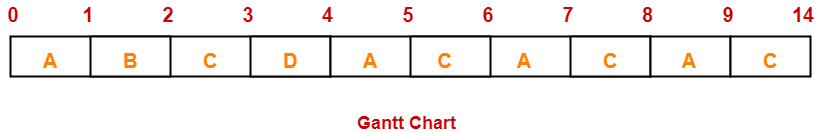Clearly, completion time of process A = 9 unit.

Thus, Option (D) is correct.

To gain better understanding about Round Robin Scheduling,

Watch this Video Lecture

Next Article- Priority Scheduling

Get more notes and other study material of Operating System.

Watch video lectures by visiting our YouTube channel LearnVidFun.